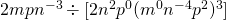Chapter 6: Polynomials

# 6.2 Negative Exponents

Consider the following chart that shows the expansion of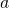for several exponents: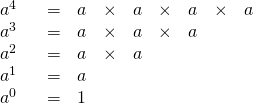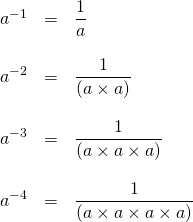If zero and negative exponents are expanded to base 2, the result is the following: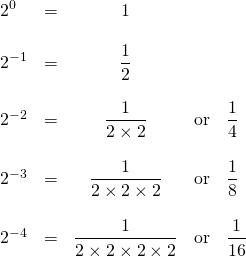The most unusual of these is the exponent 0. Any base that is not equal to zero to the zeroth exponent is always 1. The simplest explanation of this is by example.

Example 6.2.1

Simplify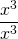.

Using the quotient rule of exponents, we know that this simplifies to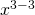, which equals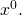And we know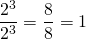,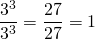,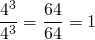,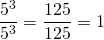,

and so on. A base raised to an exponent divided by that same base raised to that same exponent will always equal 1 unless the base is 0. This leads us to the zero power rule of exponents: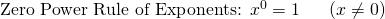This zero rule of exponents can make difficult problems elementary simply because whatever the 0 exponent is attached to reduces to 1. Consider the following examples:

Example 6.2.2

Simplify the following expressions.

1.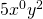Since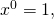this simplifies to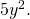2.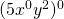Since the zero exponent is on the outside of the parentheses, everything contained inside the parentheses is cancelled out to 1.
3.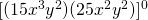Since the zero exponent is on the outside of the brackets, everything contained inside the brackets cancels out to 1.

When encountering these types of problems, always remain aware of what the zero power is attached to, since only what it is attached to cancels to 1.

When dealing with negative exponents, the simplest solution is to reciprocate the power. For instance:

Example 6.2.3

Simplify the following expressions.

1.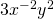Since the only negative exponent is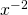, this simplifies to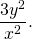2.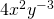Since the only negative exponent is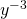, this simplifies to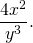3.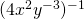Using the power of a power rule of exponents, we get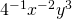.
Simplifying the negative exponents of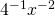, we get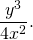4.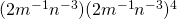First using the power of a power rule on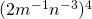yields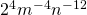.
Now we multiply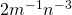by, yielding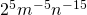.
We can write this without any negative exponents as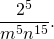# Four Rules of Negative Exponents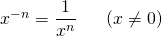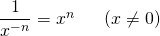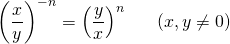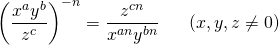# Questions

Simplify. Your answer should contain only positive exponents.

1.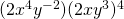2.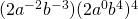3.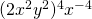4.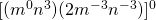5.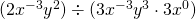6.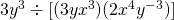7.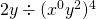8.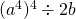9.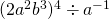10.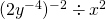11.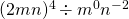12.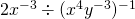13.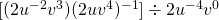14.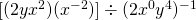15.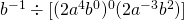16.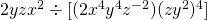17.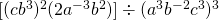18.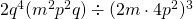19.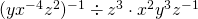20.